# Nyb F09 Unit 3

Ben
25 de Jan de 2010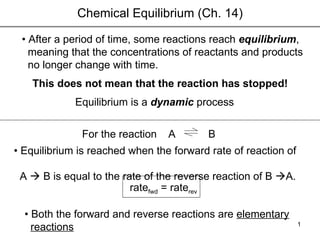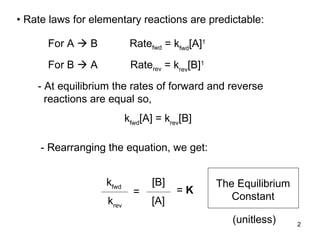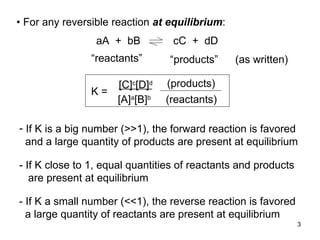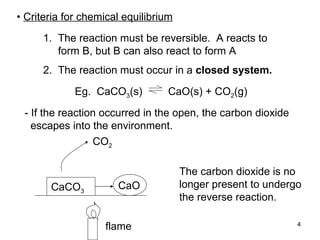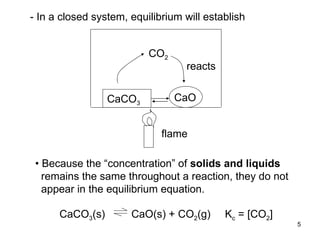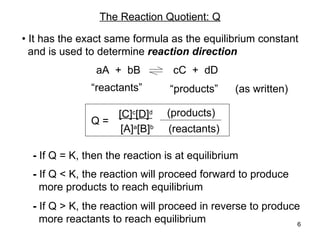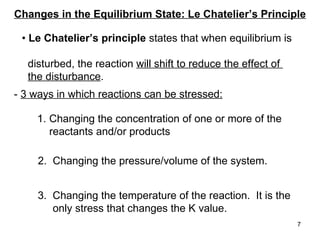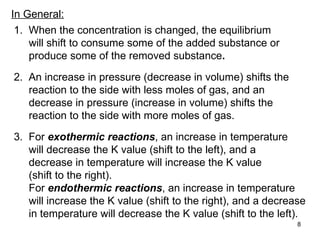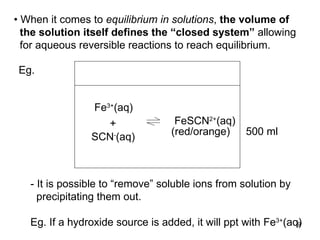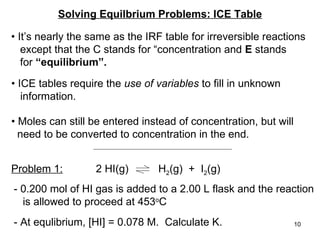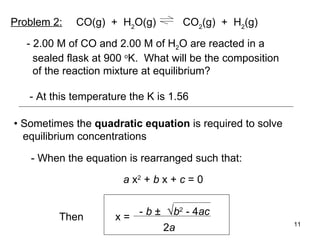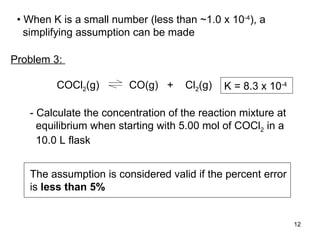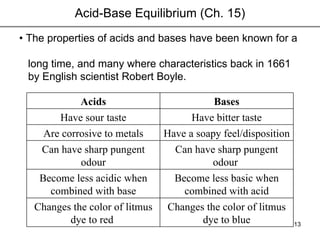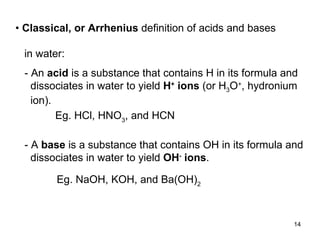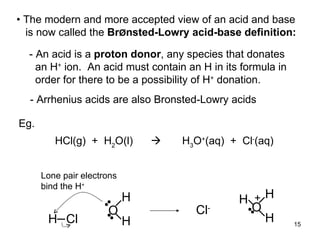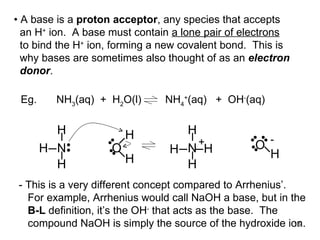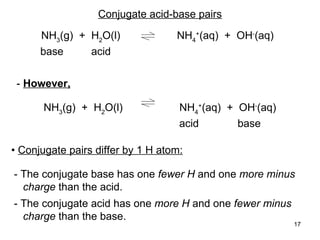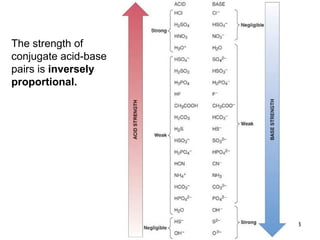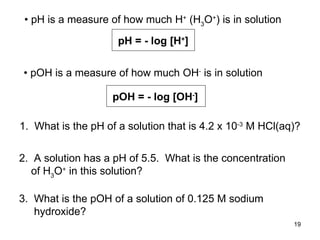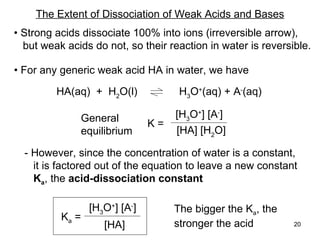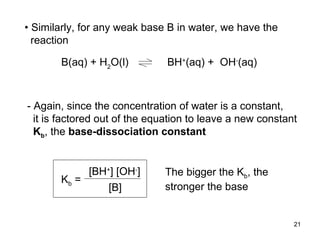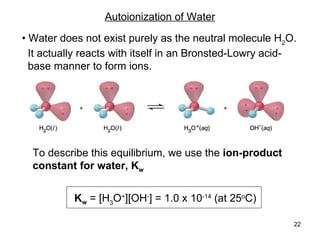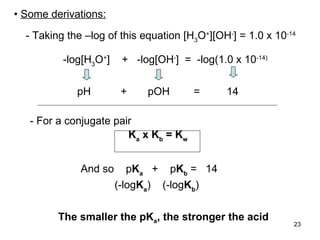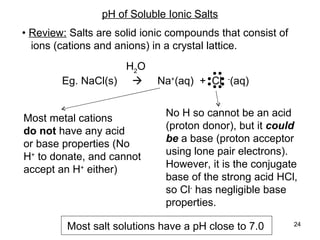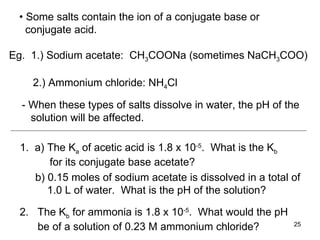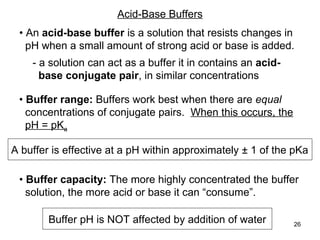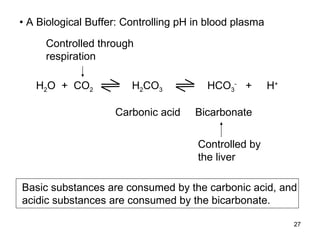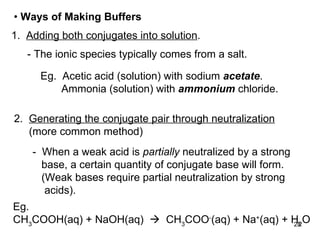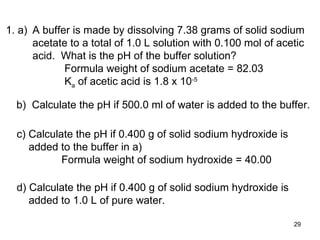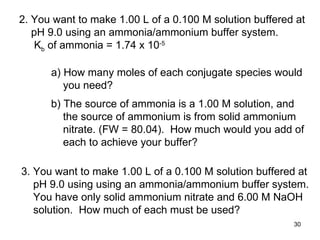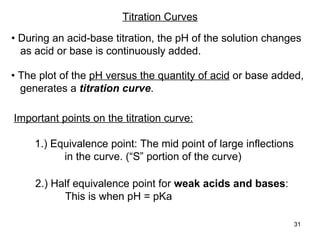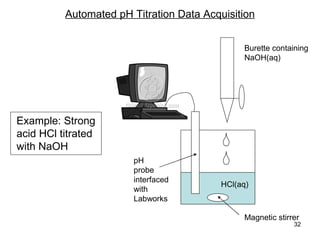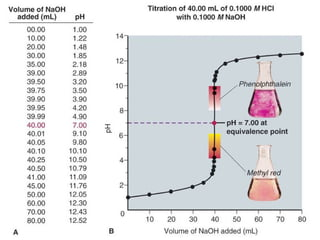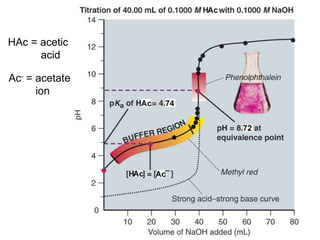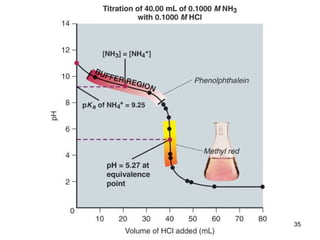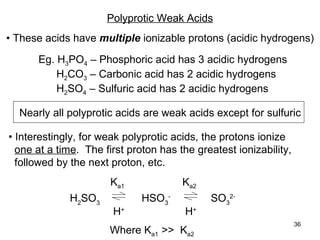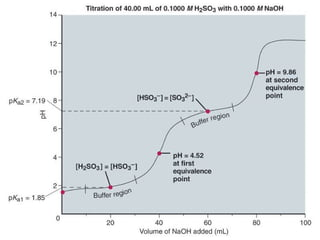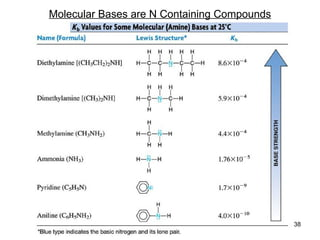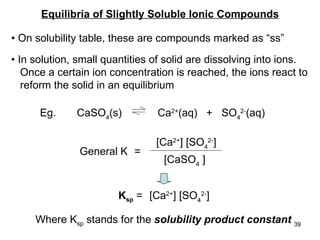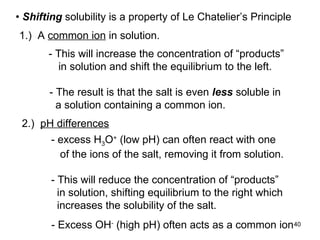1 de 40

### Nyb F09 Unit 3

• 1. Chemical Equilibrium (Ch. 14) • After a period of time, some reactions reach equilibrium , meaning that the concentrations of reactants and products no longer change with time. This does not mean that the reaction has stopped! A Equilibrium is a dynamic process B • Equilibrium is reached when the forward rate of reaction of A  B is equal to the rate of the reverse reaction of B  A. • Both the forward and reverse reactions are elementary reactions For the reaction rate fwd = rate rev
• 2. Rate fwd = k fwd [A] 1 For A  B For B  A Rate rev = k rev [B] 1 - At equilibrium the rates of forward and reverse reactions are equal so, k fwd [A] = k rev [B] - Rearranging the equation, we get: • Rate laws for elementary reactions are predictable: k fwd k rev = [B] [A] = K The Equilibrium Constant (unitless)
• 3.
• 4. • Criteria for chemical equilibrium 1. The reaction must be reversible. A reacts to form B, but B can also react to form A 2. The reaction must occur in a closed system. - If the reaction occurred in the open, the carbon dioxide escapes into the environment. The carbon dioxide is no longer present to undergo the reverse reaction. flame CaCO 3 CaO CO 2 Eg. CaCO 3 (s) CaO(s) + CO 2 (g)
• 5. • Because the “concentration” of solids and liquids remains the same throughout a reaction, they do not appear in the equilibrium equation. - In a closed system, equilibrium will establish CaCO 3 (s) CaO(s) + CO 2 (g) K c = [CO 2 ] flame CaCO 3 CaO CO 2 reacts
• 6. The Reaction Quotient: Q • It has the exact same formula as the equilibrium constant and is used to determine reaction direction - If Q = K, then the reaction is at equilibrium - If Q < K, the reaction will proceed forward to produce more products to reach equilibrium - If Q > K, the reaction will proceed in reverse to produce more reactants to reach equilibrium aA + bB cC + dD “ reactants” “ products” (as written) Q = [C] c [D] d [A] a [B] b (reactants) (products)
• 7.
• 8. In General: 1. When the concentration is changed, the equilibrium will shift to consume some of the added substance or produce some of the removed substance . 2. An increase in pressure (decrease in volume) shifts the reaction to the side with less moles of gas, and an decrease in pressure (increase in volume) shifts the reaction to the side with more moles of gas. 3. For exothermic reactions , an increase in temperature will decrease the K value (shift to the left), and a decrease in temperature will increase the K value (shift to the right). For endothermic reactions , an increase in temperature will increase the K value (shift to the right), and a decrease in temperature will decrease the K value (shift to the left).
• 9. • When it comes to equilibrium in solutions , the volume of the solution itself defines the “closed system” allowing for aqueous reversible reactions to reach equilibrium. Eg. (red/orange) - It is possible to “remove” soluble ions from solution by precipitating them out. Eg. If a hydroxide source is added, it will ppt with Fe 3+ (aq) 500 ml Fe 3+ (aq) SCN - (aq) FeSCN 2+ (aq) +
• 10. Solving Equilbrium Problems: ICE Table • It’s nearly the same as the IRF table for irreversible reactions except that the C stands for “concentration and E stands for “equilibrium”. • ICE tables require the use of variables to fill in unknown information. • Moles can still be entered instead of concentration, but will need to be converted to concentration in the end. 2 HI(g) H 2 (g) + I 2 (g) Problem 1: - At equlibrium, [HI] = 0.078 M. Calculate K. - 0.200 mol of HI gas is added to a 2.00 L flask and the reaction is allowed to proceed at 453 o C
• 11. CO(g) + H 2 O(g) CO 2 (g) + H 2 (g) - 2.00 M of CO and 2.00 M of H 2 O are reacted in a sealed flask at 900 o K. What will be the composition of the reaction mixture at equilibrium? - At this temperature the K is 1.56 • Sometimes the quadratic equation is required to solve equilibrium concentrations - When the equation is rearranged such that: a x 2 + b x + c = 0 Problem 2: - b ± √ b 2 - 4 ac 2 a x = Then
• 12. • When K is a small number (less than ~1.0 x 10 -4 ), a simplifying assumption can be made COCl 2 (g) CO(g) + Cl 2 (g) K = 8.3 x 10 -4 - Calculate the concentration of the reaction mixture at equilibrium when starting with 5.00 mol of COCl 2 in a 10.0 L flask The assumption is considered valid if the percent error is less than 5% Problem 3:
• 13. • The properties of acids and bases have been known for a long time, and many where characteristics back in 1661 by English scientist Robert Boyle. Acid-Base Equilibrium (Ch. 15) Changes the color of litmus dye to blue Changes the color of litmus dye to red Become less basic when combined with acid Become less acidic when combined with base Can have sharp pungent odour Can have sharp pungent odour Have a soapy feel/disposition Are corrosive to metals Have bitter taste Have sour taste Bases Acids
• 14. • Classical, or Arrhenius definition of acids and bases in water: - An acid is a substance that contains H in its formula and dissociates in water to yield H + ions (or H 3 O + , hydronium ion). - A base is a substance that contains OH in its formula and dissociates in water to yield OH - ions . Eg. HCl, HNO 3 , and HCN Eg. NaOH, KOH, and Ba(OH) 2
• 15. • The modern and more accepted view of an acid and base is now called the Br Ø nsted-Lowry acid-base definition: - An acid is a proton donor , any species that donates an H + ion. An acid must contain an H in its formula in order for there to be a possibility of H + donation. - Arrhenius acids are also Bronsted-Lowry acids Lone pair electrons bind the H + HCl(g) + H 2 O(l)  H 3 O + (aq) + Cl - (aq) Eg. H Cl O H H Cl - O H H H +
• 16. A base is a proton acceptor , any species that accepts an H + ion. A base must contain a lone pair of electrons to bind the H + ion, forming a new covalent bond. This is why bases are sometimes also thought of as an electron donor . - This is a very different concept compared to Arrhenius’. For example, Arrhenius would call NaOH a base, but in the B-L definition, it’s the OH - that acts as the base. The compound NaOH is simply the source of the hydroxide ion. N H H H O H H O H + N H H H H - NH 3 (aq) + H 2 O(l) NH 4 + (aq) + OH - (aq) Eg.
• 17. Conjugate acid-base pairs NH 3 (g) + H 2 O(l) NH 4 + (aq) + OH - (aq) base acid NH 3 (g) + H 2 O(l) NH 4 + (aq) + OH - (aq) - However, acid base - The conjugate base has one fewer H and one more minus charge than the acid. - The conjugate acid has one more H and one fewer minus charge than the base. • Conjugate pairs differ by 1 H atom:
• 18. The strength of conjugate acid-base pairs is inversely proportional.
• 19. • pH is a measure of how much H + (H 3 O + ) is in solution pH = - log [ H + ] 1. What is the pH of a solution that is 4.2 x 10 -3 M HCl(aq)? 2. A solution has a pH of 5.5. What is the concentration of H 3 O + in this solution? 3. What is the pOH of a solution of 0.125 M sodium hydroxide? • pOH is a measure of how much OH - is in solution pOH = - log [O H - ]
• 20. The Extent of Dissociation of Weak Acids and Bases HA(aq) + H 2 O(l) H 3 O + (aq) + A - (aq) • For any generic weak acid HA in water, we have - However, since the concentration of water is a constant, it is factored out of the equation to leave a new constant K a , the acid-dissociation constant • Strong acids dissociate 100% into ions (irreversible arrow), but weak acids do not, so their reaction in water is reversible. The bigger the K a , the stronger the acid [H 3 O + ] [A - ] [HA] [H 2 O] K = General equilibrium [H 3 O + ] [A - ] [HA] K a =
• 21. • Similarly, for any weak base B in water, we have the reaction - Again, since the concentration of water is a constant, it is factored out of the equation to leave a new constant K b , the base-dissociation constant The bigger the K b , the stronger the base B(aq) + H 2 O(l) BH + (aq) + OH - (aq) [BH + ] [OH - ] [B] K b =
• 22. Autoionization of Water • Water does not exist purely as the neutral molecule H 2 O. It actually reacts with itself in an Bronsted-Lowry acid- base manner to form ions. To describe this equilibrium, we use the ion-product constant for water, K w K w = [H 3 O + ][OH - ] = 1.0 x 10 -14 (at 25 o C)
• 23. • Some derivations: - Taking the –log of this equation [H 3 O + ][OH - ] = 1.0 x 10 -14 pH + pOH = 14 -log [H 3 O + ] + -log[ OH - ] = -log( 1.0 x 10 -14) K a x K b = K w - For a conjugate pair And so p K a + p K b = 14 (-log K a ) (-log K b ) The smaller the pK a , the stronger the acid
• 24. pH of Soluble Ionic Salts • Review: Salts are solid ionic compounds that consist of ions (cations and anions) in a crystal lattice. Most salt solutions have a pH close to 7.0 Most metal cations do not have any acid or base properties (No H + to donate, and cannot accept an H + either) No H so cannot be an acid (proton donor), but it could be a base (proton acceptor using lone pair electrons). However, it is the conjugate base of the strong acid HCl, so Cl - has negligible base properties. Eg. NaCl(s)  Na + (aq) + Cl - (aq) H 2 O
• 25. • Some salts contain the ion of a conjugate base or conjugate acid. Eg. 1.) Sodium acetate: CH 3 COONa (sometimes NaCH 3 COO) 2.) Ammonium chloride: NH 4 Cl - When these types of salts dissolve in water, the pH of the solution will be affected. 1. a) The K a of acetic acid is 1.8 x 10 -5 . What is the K b for its conjugate base acetate? b) 0.15 moles of sodium acetate is dissolved in a total of 1.0 L of water. What is the pH of the solution? 2. The K b for ammonia is 1.8 x 10 -5 . What would the pH be of a solution of 0.23 M ammonium chloride?
• 26. Acid-Base Buffers • An acid-base buffer is a solution that resists changes in pH when a small amount of strong acid or base is added. - a solution can act as a buffer it in contains an acid- base conjugate pair , in similar concentrations • Buffer range: Buffers work best when there are equal concentrations of conjugate pairs. When this occurs, the pH = pK a A buffer is effective at a pH within approximately ± 1 of the pKa • Buffer capacity: The more highly concentrated the buffer solution, the more acid or base it can “consume”. Buffer pH is NOT affected by addition of water
• 27. H 2 O + CO 2 H 2 CO 3 HCO 3 - + H + Carbonic acid Bicarbonate • A Biological Buffer: Controlling pH in blood plasma Controlled through respiration Controlled by the liver Basic substances are consumed by the carbonic acid, and acidic substances are consumed by the bicarbonate.
• 28. • Ways of Making Buffers 1. Adding both conjugates into solution . 2. Generating the conjugate pair through neutralization (more common method) - The ionic species typically comes from a salt. Eg. Acetic acid (solution) with sodium acetate . Ammonia (solution) with ammonium chloride. - When a weak acid is partially neutralized by a strong base, a certain quantity of conjugate base will form. (Weak bases require partial neutralization by strong acids). Eg. CH 3 COOH(aq) + NaOH(aq)  CH 3 COO - (aq) + Na + (aq) + H 2 O
• 29. A buffer is made by dissolving 7.38 grams of solid sodium acetate to a total of 1.0 L solution with 0.100 mol of acetic acid. What is the pH of the buffer solution? Formula weight of sodium acetate = 82.03 K a of acetic acid is 1.8 x 10 -5 1. a) b) Calculate the pH if 500.0 ml of water is added to the buffer. c) Calculate the pH if 0.400 g of solid sodium hydroxide is added to the buffer in a) Formula weight of sodium hydroxide = 40.00 d) Calculate the pH if 0.400 g of solid sodium hydroxide is added to 1.0 L of pure water.
• 30. 2. You want to make 1.00 L of a 0.100 M solution buffered at pH 9.0 using an ammonia/ammonium buffer system. K b of ammonia = 1.74 x 10 -5 a) How many moles of each conjugate species would you need? b) The source of ammonia is a 1.00 M solution, and the source of ammonium is from solid ammonium nitrate. (FW = 80.04). How much would you add of each to achieve your buffer? 3. You want to make 1.00 L of a 0.100 M solution buffered at pH 9.0 using using an ammonia/ammonium buffer system. You have only solid ammonium nitrate and 6.00 M NaOH solution. How much of each must be used?
• 31. Titration Curves • During an acid-base titration, the pH of the solution changes as acid or base is continuously added. • The plot of the pH versus the quantity of acid or base added, generates a titration curve . Important points on the titration curve: 1.) Equivalence point: The mid point of large inflections in the curve. (“S” portion of the curve) 2.) Half equivalence point for weak acids and bases : This is when pH = pKa
• 32. Automated pH Titration Data Acquisition Example: Strong acid HCl titrated with NaOH
• 33.
• 34. HAc = acetic acid Ac - = acetate ion
• 35.
• 36. Polyprotic Weak Acids • These acids have multiple ionizable protons (acidic hydrogens) Eg. H 3 PO 4 – Phosphoric acid has 3 acidic hydrogens H 2 CO 3 – Carbonic acid has 2 acidic hydrogens H 2 SO 4 – Sulfuric acid has 2 acidic hydrogens • Interestingly, for weak polyprotic acids, the protons ionize one at a time . The first proton has the greatest ionizability, followed by the next proton, etc. H 2 SO 3 HSO 3 - SO 3 2- K a1 K a2 Where K a1 >> K a2 Nearly all polyprotic acids are weak acids except for sulfuric H + H +
• 37.
• 38. Molecular Bases are N Containing Compounds
• 39. Equilibria of Slightly Soluble Ionic Compounds • On solubility table, these are compounds marked as “ss” Eg. CaSO 4 (s) Ca 2+ (aq) + SO 4 2- (aq) • In solution, small quantities of solid are dissolving into ions. Once a certain ion concentration is reached, the ions react to reform the solid in an equilibrium General K [ Ca 2+ ] [ SO 4 2- ] [ CaSO 4 ] = K sp = [ Ca 2+ ] [ SO 4 2- ] Where K sp stands for the solubility product constant
• 40. • Shifting solubility is a property of Le Chatelier’s Principle - This will increase the concentration of “products” in solution and shift the equilibrium to the left. - The result is that the salt is even less soluble in a solution containing a common ion. 2.) pH differences - excess H 3 O + (low pH) can often react with one of the ions of the salt, removing it from solution. - This will reduce the concentration of “products” in solution, shifting equilibrium to the right which increases the solubility of the salt. - Excess OH - (high pH) often acts as a common ion 1.) A common ion in solution.

### Notas do Editor

1. An non-chemical analogy for an equilibrium situation is with population. Say the island of Montreal has a population of 2.5 million people, and this number hasn’t changed for the last 3 years. Intuitively, you probably realize that the reason the number hasn’t change is not because no one is moving or no one is dying or being born. It is sure that these things are still happening. If so, how does the population remain “unchanging”? The factors that would reduce population (death and moving out of the island) must be balanced by the factors that increase population (birth and moving into the island). Another way to put it is that equilibrium is created from a balance of rates. Rate of loss of population = Rate of gain When it comes to chemical equilibrium, the concept is very similar. The rate of the forward reaction is equal to the rate of the reverse reaction, creating a particular ratio of products to reactants at any given time.
2. This slide essentially explains how the K equilibrium constant is established: it’s the ratio of the forward rate constant over the reverse rate constant, OR more simply, the concentration of products over the concentration of reactants.
3. Keep in mind that the reactions are reversible, and can actually be written the other way. This makes the K of the reverse reaction (call it Krev) the inverse of Kfor. For example, if the K for a particular reaction is 25.0. The K for the reverse reaction is 1/25.0 = 0.04.
4. Note that in the textbook, they refer to the equilibrium constant as Kc, where the subscript c stands for concentration. So for the reaction of calcium carbonate, the equilibrium constant would be: Kc = [CO2] ONLY SUBSTANCES THAT CAN CHANGE IN CONCENTRATION AFFECT EQUILIBRIUM. Concentrations of solids and liquids don’t change since the substance itself defines the volume . Take 1.0 L of liquid water. It weighs 1000 grams, which equals 55.56 moles. This many moles in 1.0 L gives water a concentration of 55.56 M. What happens if we boil away 500.0 ml of water? What will be the concentration now? As you can see, we’ve reduced the moles of water by half. However, we’ve also reduced its volume by half! The liquid water is still 55.56 M, and will always be this concentration regardless of quantity.
5. Calculating Q is exactly the same as the definition of the equilibrium constant K. Q is used when the chemical situation may not be at equilibrium. When Q is compared to K, we can see how the reaction will change over time to achieve equilibrium. Example: Problem 17.5 For the reaction N2O4(g) 2 NO2(g), the K = 0.21. You add 0.12 mol of N2O4 and 0.55 mol of NO2 into a 1.0 L flask and seal it. Is the reaction at equilibrium? If not, which way will the reaction proceed to reach equilibrium? Q = 0.552/0.12 = 2.5 This number is much bigger than K. Equilibrium will be established when more N2O4 reactant is produced. Therefore, the reaction will proceed right (to make more reactant) over time to reach equilibrium.
6. For the reaction PCl3(g) + Cl2(g) PCl5(g) We can see that the K for this reaction is = [PCl5]/[PCl3][Cl2], and let’s pretend that the K is 1.0 to make the math easier Changes in concentration What would happen to this reaction if all of a sudden we added more PCl3(g) to the reaction system? In general, the equilibrium system reacts to consume some of the added substance or produce some of the removed substance. Since we added reactant the reaction will shift to the right to try to consume it (get rid of the excess). Let’s use some simple numbers to see what this sudden stress on the system does. Say the concentration of all three compounds in the above reaction is 1.0 M. PCl3(g) + Cl2(g) PCl5(g) At equilibrium: 1.0 M 1.0 M 1.0 M making K = 1 Now let’s say that the injection of extra PCl3 has increased its concentration to 2.0 M. What has happened to the ratio of products to reactants? We can use reaction quotient, Q to help us predict the shift in equilibrium. Q=[PCl5][PCl3] [Cl2] Q=1.0 M(2.0 M)(1.0 M) = 0.50 We can see that Q &lt; K. This means the top number is too small. The reaction needs to make more product such that Q can equal K. This is the mathematical explanation for the system shifting to the right to try to consume excess added substance. Changes in concentration can also be achieved by removal of a reactant or product. Let’s say Cl2(g) was able to be removed from the reaction mixture, reducing its concentration. The reaction will shift to the left, to produce more of what’s been removed. The effect of changing pressure (by changing total volume) Of course, pressure changes will have an effect only on gases, since solids and liquids are incompressible. Let’s see what happens to the same reaction as above in a system where we can alter to total pressure. Movable pistonHigh pressure (small volume)Reaction in Equilibrium 1.0 LLow pressure (Large volume) 2.0 L 0.50 L Say we have these quantities of gases in 1.0 L volume PCl3(g) + Cl2(g) PCl5(g) 1.0 mol 1.0 mol 1.0 mol 1.0 M 1.0 M 1.0 M Let’s see what would happen to the system if the piston moved down, reducing the volume to 0.50 L. There is still 1.0 mol of each substance but now in a new volume of 0.50 L, all the concentrations have become 2.0 M.Q=2.0 M(2.0 M)(2.0 M) = 0.50 Q &lt; K so the reaction shifts to the right to form more products, essentially reducing total gas volume and restoring the pressure. Another way to look at it is that there are 2 molar equivalents of gas on the reactant side, whereas there’s only 1 molar equivalent of gas on the product side. An increase in pressure (decrease in volume) shifts the reaction to the side with less moles of gas. Now if the piston is moved up to make the volume 2.0 L, all the concentrations become 0.50 M.Q=0.50 M(0.50 M)(0.50 M) = 2.0 Q &gt; K so the reaction shifts to the left to form more reactants. An decrease in pressure (increase in volume) shifts the reaction to the side with more moles of gas. What would happen in the same piston-vessel above with this reaction: H2(g) + I2(g) 2HI(g) 2 mole gas 2 mol gas In terms of pressure, there is no way that the reaction could shift to relieve the pressure, so nothing happens. In terms of concentrations, you can see that all the concentration changes will occur in the same proportions of product to reactant, so the K value has not changed. Therefore, if both sides of the equation have an equal number of moles, the reaction does not shift. The effect of changes in temperature To know how a change in temperature will affect the equilibrium of a reaction, we have to know the enthalpy of the reaction, ΔH , or the heat involved with the reaction process. If the ΔH is negative, the reaction is exothermic and releases energy into the surrounding environment during the reaction. If the ΔH is positive, the reaction is endothermic and requires energy to occur. The energy the reaction requires needs to be drawn from the surrounding environment for it to occur. PCl3(g) + Cl2(g) PCl5(g) ΔH0rxn = -111 kJ/mole The forward reaction is a favorable one that will release heat. What happens if we increase the temperature of this reaction? Since release of heat is a requirement for the reaction, increasing the temperature hinders the heat loss and will slow down the forward reaction and favor the back reaction . However, if we lowered the temperature, the loss of heat from the reaction will occur more readily, and therefore the forward reaction will occur to a greater extent. The exact opposite happens for an endothermic reaction. The reaction requires heat energy to occur, so increasing the temperature favors the forward reaction, and reducing the temperature reduces the forward reaction. The difference with the temperature effect is that the favorability of the reaction to form the products or reactants has been altered, and in doing so changes the concentrations of the reactants and products at a new equilibrium position, thereby changing the K value . It is the only factor that changes the K value of the reaction. Changes in concentration and changes in pressure do not change the K value. For exothermic reactions, an increase in temperature will decrease the K value (shift to the left), and a decrease in temperature will increase the K value (shift to the right). For endothermic reactions, an increase in temperature will increase the K value (shift to the right), and a decrease in temperature will decrease the K value (shift to the left).
7. This example is done using concentration in the IRE table. Concentration (M) 2HI(g) H2(g) + I2(g) Initial I: 0.100 0 0 Reaction R: -2x +x +x Equilibrium E: 0.100-2x x x Since the reactants are initially at 0, it is for certain that the reaction will proceed to the right to achieve equilibrium. This is why the reactant is decreasing by 2x, whereas the products are increasing by x according to the stoichiometry. The E line represents the concentrations of the entire reaction composition at equilibrium. Therefore, we need to solve for x.
8. x = 1.11 M and now all concentrations can be calculated. Try the same question except starting with 2.00 M CO(g) and 1.00 M H2O 0.56 x2 – 4.68 x + 3.12 = 0 To solve for x, you have no choice but to use the quadratic formula. x = 7.6 M and/or 0.73 M. However, the 7.6 M doesn’t work since it results in a negative concentration for CO and H2O.
9. The qualification of a small K is approximate. It also depends on the quantity of the reactants. The fact that K is small means that when equilibrium is established, there is a very low quantity of products compared to reactants. The forward reaction is not favored. Concentration (M) COCl2(g) CO(g) + Cl2(g) Initial I: 0.500 0 0 Reaction R: -x +x +x Equilibrium E: 0.500-x x x The assumption: since K is small, x will also be very small such that 0.500-x ~ 0.500. We are assuming that x is negligible, kind of like 1 million dollars minus 1 dollar. It’s still essentially 1 million dollars. Now the K equilibrium expression will be simplified and we can avoid using the quadratic formula to solve x. Solving for x gives 0.0204. Now, is 0.0204 really small enough such that 0.500-0.0204 is considered ~0.500? The assumption is valid within 5% error so… Therefore the assumption is valid.
10. The classical definition is the most obvious way to think of acids and bases. However, the definition has a flaw when it comes to defining a weak base. For example, what is NH3? An acid or a base? According to the classical definition above, if it is not a neutral compound, NH3 looks as though it could be an acid since it contains H in its formula. But by all observable properties ( slide 2 ), it is clearly a base, yet it does not contain OH in its formula. How can this be?
11. Clearly, a new concept had to be developed to define a compound such as NH3 as a base. TWO THINGS TO NOTE: 1.) The terms “proton” and “hydrogen ion, H+” mean the same thing and often times are used interchangeably. Why is the H+ ion called a proton? Hydrogen is a unique element in that it has only one proton in its nucleus and no neutrons. The H atom also has only one electron. The H+ ion is created when the hydrogen atom loses an electron. What does the H+ ion have left? Just a single proton: the H+ ion is essentially nothing more than a single proton! 2.) A lone pair of electrons surrounding an atom are valance electrons that complete the atom’s octet, but are NOT involved in covalent bonding.
12. you can see why NH3 is a base: when added to water, it produces OH- in solution.
13. Notice that the reaction is reversible. When the reaction goes backwards, we have new chemical species that also have an acid and base, as defined by the Bronsted-Lowry definition. Therefore, NH3 and NH4+ are considered a conjugate pair, as well as H2O and OH-. Exercise: match up conjugate pairs from the following list: Cl-, OH-, CH3COOH, NH4+, H2SO4, H3O+, CH3COO-, NH3, H2O, SO42-, HCl, Na+, HSO4-
14. Calculating the extent of dissociation of acids and bases For acids and bases, the amount of H3O+ and OH- they can produce in solution indicate how strong of an acid or base they can be respectively. In chemistry, HCl is often used as your model strong acid. The model weak acid used is often acetic acid, CH3COOH. Because we know that strong acids dissociate 100% into ions, it is easy to determine how much H3O+ is produced given the concentration of the acid. A 0.10 M solution of HCl would react as follows: HCl(aq) + H2O(l)  H3O+(aq) + Cl-(aq) 0.10 M 0.10 M 0.10 M According to the equation, we have proof that HCl dissociates 100% into ions The pH of a 0.10 M solution of HCl(aq) would be 1.0 How much H3O+ is produced from a 0.10 M solution of acetic acid? What would be the pH of the solution? Since this is a reversible reaction in solution, the reaction will reach equilibrium, and the extent of the forward reaction to produce products will be dependent on its acid-dissociation constant Ka. For acetic acid, Ka = 1.8 x 10-5 CH3COOH(aq) + H2O(l) H3O+(aq) + CH3COO-(aq) I 0.10 M R -x +x +x E 0.10 M x x By solving for x, we will know the concentration of H3O+ produced from a 0.10 M solution of acetic acid. At first glance, it looks like we will have to solve a quadratic formula after cross multiplying. However, what do you notice about the value for Ka? It is a very small number, meaning that the forward reaction does not proceed to a great extent. Therefore, we can assume that x will be small such that 0.10 – x ~ 0.10 The equation simplifies to: Now solving for x is simple: x2 = 1.8 x 10-6. x = 1.3 x 10-3 M = [H3O+]= [CH3COO-] pH = 2.9 Using the formula above, we get a percent dissociation of only 1.34 %.
15. Since self-ionization is an inherent property of water as defined by the Kw expression, it means that there is always going to be H3O+ ion and OH- ions in dilute aqueous solutions . This concept is very important for you to understand. The concentrations of these ions however, will be changed by addition of either acid or base to water. Question: A 0.01 M solution of HCl is made. What is the concentration of OH- in this solution? 0.01 M of HCl will generate 0.01 M of H3O+ (100% ionization). Using the Kw equation, we get: [OH-] = Kw/[H3O+] = 1 x 10-14/0.01 M = 1 x 10-12 M. The OH- concentration is extremely small, but there is still some in solution.
16. Proof of the bottom half of the slide: So for any generic acid HA in water we have the reaction: HA(aq) + H2O(l) H3O+(aq) + A-(aq) Ka = [H3O+][A-] [HA] A- is the conjugate base of the acid HA. A- will therefore act as a base in solution. The reaction of the conjugate base A- in water gives: A-(aq) + H2O(l) OH-(aq) + HA(aq) Kb = [OH-][HA] [A-] When we multiply the two equilibrium constants: Ka x Kb = [H3O+][A-] x [OH-][HA] [HA] x [A-] You can see that the same species in both the numerator and denominator cancel out, leaving behind: Ka x Kb = [H3O+][OH-] = Kw Analogous to the invention of the pH scale for convenience, we can also represent the Ka and Kb values in numbers that are easier to work with. These are the pKa and pKb. pKa = -logKa and pKb = -logKb
17. 1. a) Kb (of acetate) = Kw/Ka (of acetic acid) Kb = 5.56 x 10-10 b) Dissolving 0.15 mol of sodium acetate will produce 0.15 mol of acetate ion. NaCH3COO(aq)  Na+(aq) + CH3COO-(aq) 0.15 mol 0.15 mol 0.15 mol The acetate ion will react with water reversibly and create an equilibrium CH3COO-(aq) + H2O(l) CH3COOH(aq) + OH-(aq) I 0.15 M excess 0 0 R -x +x +x E 0.15 – x x x Since the Kb is super small, x will be extremely small such that 0.15 – x ~ 0.15 Now the equilibrium equation becomes 5.56 x 10-10 = x2/0.15 Solving for x gives 9.13 x 10-6 M, which happens to be the concentration of OH- in the sodium acetate solution. Since Kw = [H3O+][OH-], solving for [H3O+] gives 1.10 x 10-9 M. Therefore pH of the sodium acetate salt solution = 8.96 2.) pH of the ammonium chloride solution = 4.95
18. A particular acid-base buffer system is effective at resisting pH changes with a certain pH range, also called the buffer range . If there are equal quantities of acid and conjugate base for a buffer… Ka = [H3O+][A-] If [A-] and [HA] are the same number, you can see that they will cancel out and [HA] Ka = [H3O+] When taking the –log of both sides of the equation, pKa = pH. Say you made an acetic acid/acetate buffer solution. At what pH is the buffer most effective? Acetic acid has a Ka = 1.8 x 10-5, so it’s pKa is 4.7. Therefore, an acetic acid/acetate buffer solution is most capable of resisting pH changes in the range of 4.7 plus or minus 1 pH value. Capacity. Eg a 1.0 M buffer can neutralize more than a 0.10 M buffer.
19. The buffer concept is extremely important in biology. Molecules such as enzymes, proteins, DNA, fats, etc. within the cells of living organisms function optimally at near neutral pH. Slight fluctuations in pH could destroy molecular function, leading to death of the cell, and eventual death of the organism. Therefore, it is absolutely crucial for cellular fluids to maintain a particular pH. Shown in the slide are the chemical components that regulate blood pH to keep it very close to 7.4. This is the main buffer system that soaks up lactic acid produced by muscle cells during vigorous (anaerobic) exercise. It is believed that one of the main reasons vigorous activity cannot be sustained indefinitely is because of the lowering of pH by production of excess lactic acid during vigorous exercise. If you take a break, allow the buffer system to restore the pH balance, another burst of vigorous activity can be achieved.
20. Method #1 is fairly obvious. Since we need both the acid and conjugate base in the same solution, we simply find chemicals that contains both sources. For example, to make an acetic acid/acetate buffer, we would simply find some acetic acid solution, and a salt that contains acetate ion, such as sodium acetate. Method #2 is equally common to use. Say we have 1.0 mol of acetic acid, but no sodium acetate. The acetate ion can be generated by reacting with NaOH. Acetic acid and sodium hydroxide will neutralize each other to produce a salt and water. However, we don’t want to neutralize all the acetic acid. Optimally, we would neutralize about half the quantity of the acid. CH3COOH(aq) + NaOH(aq) CH3COO-(aq) + Na+(aq) + H2O I 1.0 mol 0.50 mol 0 0 0 R -0.50 mol -0.50 mol +0.50 +0.50 +0.50 F 0.50 mol 0 0.50 mol 0.50 mol 0.50 mol Now with equal quantities of acid and conjugate base in solution, an equilibrium will be achieved to create a buffer.